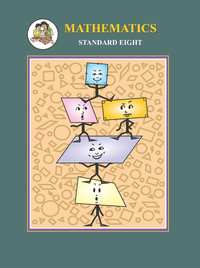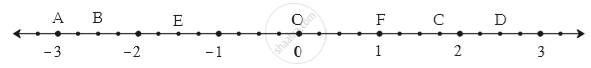Share

# Balbharati solutions for Maharashtra state board (SSC) Class 8 Mathematics chapter 1 - Rational and Irrational numbers [Latest edition]

Textbook page

#### Chapters## Chapter 1: Rational and Irrational numbers

Practice Set 1.1Practice Set 1.2Practice Set 1.3Practice Set 1.4

### Balbharati solutions for Maharashtra state board (SSC) Class 8 Mathematics Chapter 1 Rational and Irrational numbers Practice Set 1.1 [Page 2]

Practice Set 1.1 | Q 1.1 | Page 2

Show the following numbers on a number line. Draw a separate number line for each example.

3/2 , 5/2 , -3/2

Practice Set 1.1 | Q 1.2 | Page 2

Show the following numbers on a number line. Draw a separate number line for each example.

7/5 , (-2)/5 , (-4)/5

Practice Set 1.1 | Q 1.3 | Page 2

Show the following numbers on a number line. Draw a separate number line for each example.

(-5)/8 , 11/8

Practice Set 1.1 | Q 1.4 | Page 2

Show the following numbers on a number line. Draw a separate number line for each example.

13/10 , (-17)/10

Practice Set 1.1 | Q 2 | Page 2

Observe the number line and answer the questions.(1) Which number is indicated by point B?

(2) Which point indicates the number 1 3/4 ?

(3) State whether the statement, ‘the point D denotes the number 5/2’ is true or false.

### Balbharati solutions for Maharashtra state board (SSC) Class 8 Mathematics Chapter 1 Rational and Irrational numbers Practice Set 1.2 [Page 3]

Practice Set 1.2 | Q 1 | Page 3

Compare the following number.

-7,-2

Practice Set 1.2 | Q 2 | Page 3

Compare the following number.

0,(-9)/5

Practice Set 1.2 | Q 3 | Page 3

Compare the following number.

8/7,0

Practice Set 1.2 | Q 4 | Page 3

Compare the following number.

(-5)/4,1/4

Practice Set 1.2 | Q 5 | Page 3

Compare the following number.

40/29,141/29

Practice Set 1.2 | Q 6 | Page 3

Compare the following number.

-17/20,(-13)/20

Practice Set 1.2 | Q 7 | Page 3

Compare the following number.

15/12,7/16

Practice Set 1.2 | Q 8 | Page 3

Compare the following number.

(-25)/8,(-9)/4

Practice Set 1.2 | Q 9 | Page 3

Compare the following number.

12/15,3/5

Practice Set 1.2 | Q 10 | Page 3

Compare the following number.

(-7)/11,(-3)/4

### Balbharati solutions for Maharashtra state board (SSC) Class 8 Mathematics Chapter 1 Rational and Irrational numbers Practice Set 1.3 [Page 4]

Practice Set 1.3 | Q 1 | Page 4

Write the following rational number in decimal form.

9/37

Practice Set 1.3 | Q 2 | Page 4

Write the following rational number in decimal form.

18/42

Practice Set 1.3 | Q 3 | Page 4

Write the following rational number in decimal form.

9/14

Practice Set 1.3 | Q 4 | Page 4

Write the following rational number in decimal form.

(-103)/5

Practice Set 1.3 | Q 5 | Page 4

Write the following rational number in decimal form.

-11/13

### Balbharati solutions for Maharashtra state board (SSC) Class 8 Mathematics Chapter 1 Rational and Irrational numbers Practice Set 1.4 [Pages 5 - 6]

Practice Set 1.4 | Q 1 | Page 5

The number sqrt2 is shown on a number line. Steps are given to show sqrt3 on the number line using sqrt2. Fill in the boxes properly and complete the activity.

Activity :

• The point Q on the number line shows the number ...... .
• A line perpendicular to the number line is drawn through the point Q. Point R is at unit distance from Q on the line.
• Right angled  ORQ is obtained by drawing seg OR.

l  (OQ) = sqrt2 ,    l(QR) = 1

therefore by Pythagoras theorem,

[l(OR)]^2  = [l(OQ)]^2 + [l(QR)]^2

= _____2 + _____2 = _____ + _____

= ______

∴ l(OR) = _____

Draw an arc with centre O and radius OR. Mark the point of intersection of the line and the arc as C. The point C shows the number √3.

Practice Set 1.4 | Q 2 | Page 6

Show the number √5 on the number line.

Practice Set 1.4 | Q 3 | Page 6

Show the number √7 on the number line.

## Chapter 1: Rational and Irrational numbers

Practice Set 1.1Practice Set 1.2Practice Set 1.3Practice Set 1.4## Balbharati solutions for Maharashtra state board (SSC) Class 8 Mathematics chapter 1 - Rational and Irrational numbers

Balbharati solutions for Maharashtra state board (SSC) Class 8 Mathematics chapter 1 (Rational and Irrational numbers) include all questions with solution and detail explanation. This will clear students doubts about any question and improve application skills while preparing for board exams. The detailed, step-by-step solutions will help you understand the concepts better and clear your confusions, if any. Shaalaa.com has the Maharashtra State Board Maharashtra state board (SSC) Class 8 Mathematics solutions in a manner that help students grasp basic concepts better and faster.

Further, we at Shaalaa.com provide such solutions so that students can prepare for written exams. Balbharati textbook solutions can be a core help for self-study and acts as a perfect self-help guidance for students.

Concepts covered in Maharashtra state board (SSC) Class 8 Mathematics chapter 1 Rational and Irrational numbers are To Show Rational Numbers on a Number Line, Comparison of Rational Numbers, Decimal Representation of Rational Numbers, Concept of Irrational Numbers.

Using Balbharati Class 8 solutions Rational and Irrational numbers exercise by students are an easy way to prepare for the exams, as they involve solutions arranged chapter-wise also page wise. The questions involved in Balbharati Solutions are important questions that can be asked in the final exam. Maximum students of Maharashtra State Board Class 8 prefer Balbharati Textbook Solutions to score more in exam.

Get the free view of chapter 1 Rational and Irrational numbers Class 8 extra questions for Maharashtra state board (SSC) Class 8 Mathematics and can use Shaalaa.com to keep it handy for your exam preparation